# Adjoint representation of a Lie group

(diff) ← Older revision | Latest revision (diff) | Newer revision → (diff)

or algebraic groupThe linear representation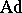ofin the tangent space(or in the Lie algebraof) mapping eachto the differential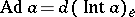of the inner automorphism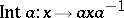. If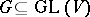is a linear group in a space, thenThe kernel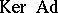contains the centre of, and ifis connected and if the ground field has characteristic zero, coincides with this centre. The differential of the adjoint representation ofatcoincides with the adjoint representation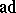of.

The adjoint representation of a Lie algebrais the linear representationof the algebrainto the moduleacting by the formula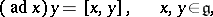whereis the bracket operation in the algebra. The kernelis the centre of the Lie algebra. The operators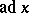are derivations ofand are called inner derivations. The image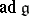is called the adjoint linear Lie algebra and is an ideal in the Lie algebra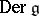of all derivations of, moreover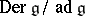is the one-dimensional cohomology space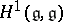of, defined by the adjoint representation. In particular,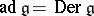ifis a semi-simple Lie algebra over a field of characteristic zero.

How to Cite This Entry: